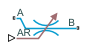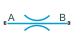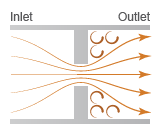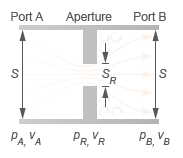# Local Restriction (2P)

Restriction in flow area in two-phase fluid network

•Libraries:
Simscape / Foundation Library / Two-Phase Fluid / Elements

## Description

The Local Restriction (2P) block models the pressure drop due to a localized reduction in flow area, such as a valve or an orifice, in a two-phase fluid network.

Ports A and B represent the restriction inlet and outlet. The input physical signal at port AR specifies the restriction area. Alternatively, you can specify a fixed restriction area as a block parameter.

The block icon changes depending on the value of the Restriction type parameter.

Restriction TypeBlock Icon

`Variable``Fixed`The restriction is adiabatic. It does not exchange heat with the environment.

The restriction consists of a contraction followed by a sudden expansion in flow area. The fluid accelerates during the contraction, which causes the pressure to drop. In the expansion zone, if the Pressure recovery parameter is `off`, the momentum of the accelerated fluid is lost. If the Pressure recovery parameter is `on`, the sudden expansion recovers some of the momentum and allows the pressure to rise slightly after the restriction.

Local Restriction Schematic### Mass Balance

The mass balance equation is

`${\stackrel{˙}{m}}_{A}+{\stackrel{˙}{m}}_{B}=0,$`

where:

• ${\stackrel{˙}{m}}_{A}$ and ${\stackrel{˙}{m}}_{B}$ are the mass flow rates into the restriction through port A and port B.

### Energy Balance

The energy balance equation is

`${\varphi }_{A}+{\varphi }_{B}=0,$`

where ϕA and ϕB are the energy flow rates into the restriction through port A and port B.

The local restriction is assumed to be adiabatic, and therefore, the change in specific total enthalpy is zero. At port A,

`${u}_{A}+{p}_{A}{\nu }_{A}+\frac{{w}_{A}^{2}}{2}={u}_{R}+{p}_{R}{\nu }_{R}+\frac{{w}_{R}^{2}}{2},$`

while at port B,

`${u}_{B}+{p}_{B}{\nu }_{B}+\frac{{w}_{B}^{2}}{2}={u}_{R}+{p}_{R}{\nu }_{R}+\frac{{w}_{R}^{2}}{2},$`

where:

• uA, uB, and uR are the specific internal energies at port A, at port B, and the restriction aperture.

• pA, pB, and pR are the pressures at port A, port B, and the restriction aperture.

• νA, νB, and νR are the specific volumes at port A, port B, and the restriction aperture.

• wA, wB, and wR are the ideal flow velocities at port A, port B, and the restriction aperture.

The block computes the ideal flow velocity as

`${w}_{A}=\frac{{\stackrel{˙}{m}}_{ideal}{\nu }_{A}}{S}$`

at port A, as

`${w}_{B}=\frac{{\stackrel{˙}{m}}_{ideal}{\nu }_{B}}{S}$`

at port B, and as

`${w}_{R}=\frac{{\stackrel{˙}{m}}_{ideal}{\nu }_{R}}{{S}_{R}},$`

inside the restriction, where:

• ${\stackrel{˙}{m}}_{ideal}$ is the ideal mass flow rate through the restriction.

• S is the flow area at port A and port B.

• SR is the flow area of the restriction aperture.

The block computes the ideal mass flow rate through the restriction as:

`${\stackrel{˙}{m}}_{ideal}=\frac{{\stackrel{˙}{m}}_{A}}{{C}_{D}},$`

where CD is the flow discharge coefficient for the local restriction.

Local Restriction Variables### Momentum Balance

The change in momentum between the ports reflects in the pressure loss across the restriction. That loss depends on the mass flow rate through the restriction, though the exact dependence varies with flow regime. When the flow is turbulent,

`$\stackrel{˙}{m}={S}_{\text{R}}\left({p}_{\text{A}}-{p}_{\text{B}}\right)\sqrt{\frac{2}{|{p}_{\text{A}}-{p}_{\text{B}}|{\nu }_{\text{R}}{K}_{\text{T}}}},$`

where KT is defined as:

`${K}_{\text{T}}=\left(1+\frac{{S}_{\text{R}}}{S}\right)\left(1-\frac{{\nu }_{\text{in}}}{{\nu }_{\text{R}}}\frac{{S}_{\text{R}}}{S}\right)-2\frac{{S}_{\text{R}}}{S}\left(1-\frac{{\nu }_{\text{out}}}{{\nu }_{\text{R}}}\frac{{S}_{\text{R}}}{S}\right),$`

in which the subscript `in` denotes the inlet port and the subscript `out` the outlet port. Which port serves as the inlet and which serves as the outlet depends on the pressure differential across the restriction. If pressure is greater at port A than at port B, then port A is the inlet; if pressure is greater at port B, then port B is the inlet.

When the flow is laminar,

`$\stackrel{˙}{m}={S}_{\text{R}}\left({p}_{\text{A}}-{p}_{\text{B}}\right)\sqrt{\frac{2}{\Delta {p}_{\text{Th}}{\nu }_{\text{R}}{\left(1-\frac{{S}_{\text{R}}}{S}\right)}^{2}},}$`

where ΔpTh denotes the threshold pressure drop at which the flow begins to smoothly transition between laminar and turbulent,

`$\Delta {p}_{\text{Th}}=\left(\frac{{p}_{\text{A}}+{p}_{\text{B}}}{2}\right)\left(1-{B}_{\text{L}}\right),$`

in which BLam is the Laminar flow pressure ratio parameter. The flow is laminar if the pressure drop from port A to port B is below the threshold value; otherwise, the flow is turbulent.

The pressure at the restriction area, pR likewise depends on the flow regime. When the flow is turbulent:

`${p}_{\text{R,L}}={p}_{\text{in}}-\frac{{\nu }_{\text{R}}}{2}{\left(\frac{\stackrel{˙}{m}}{{S}_{\text{R}}}\right)}^{2}\left(1+\frac{{S}_{\text{R}}}{S}\right)\left(1-\frac{{\nu }_{\text{in}}}{{\nu }_{\text{R}}}\frac{{S}_{\text{R}}}{S}\right).$`

When the flow is laminar:

`${p}_{\text{R,L}}=\frac{{p}_{\text{A}}+{p}_{\text{B}}}{2}.$`

### Assumptions and Limitations

• The restriction is adiabatic. It does not exchange heat with its surroundings.

## Ports

### Input

expand all

Input physical signal that controls the flow restriction area. The signal saturates when its value is outside the minimum and maximum restriction area limits, specified by the Minimum restriction area and Maximum restriction area parameters.

#### Dependencies

To enable this port, set the Restriction type parameter to `Variable`.

### Conserving

expand all

Two-phase fluid conserving port associated with the inlet or outlet of the local restriction. The block has no intrinsic directionality.

Two-phase fluid conserving port associated with the inlet or outlet of the local restriction. The block has no intrinsic directionality.

## Parameters

expand all

Whether the restriction area can change during simulation:

• `Variable` — The input physical signal at port AR specifies the restriction area, which can vary during simulation. The Minimum restriction area and Maximum restriction area parameters specify the lower and upper bounds for the restriction area.

• `Fixed` — The restriction area, specified by the Restriction area block parameter value, remains constant during simulation.

Lower bound for the restriction cross-sectional area. You can use this parameter to represent the leakage area. The input signal at port AR saturates at this value to prevent the restriction area from further decreasing.

#### Dependencies

To enable this parameter, set Restriction type to `Variable`.

Upper bound for the restriction cross-sectional area. The input signal at port AR saturates at this value to prevent the restriction area from further increasing.

#### Dependencies

To enable this parameter, set Restriction type to `Variable`.

Area normal to the flow path at the restriction.

#### Dependencies

To enable this parameter, set Restriction type to `Fixed`.

Area normal to the flow path at ports A and B. This area is assumed to be the same for both ports.

The discharge coefficient is a semi-empirical parameter commonly used to characterize the flow capacity of an orifice. This parameter represents the ratio of the actual mass flow rate through the orifice to the ideal mass flow rate.

Ratio of the outlet to the inlet port pressure at which the flow regime is assumed to switch from laminar to turbulent. The prevailing flow regime determines the equations used in simulation. If the flow is laminar, the pressure drop across the restriction is linear with respect to the mass flow rate. If the flow is turbulent, the pressure drop across the restriction is quadratic with respect to the mass flow rate.

## Version History

Introduced in R2015b

expand all